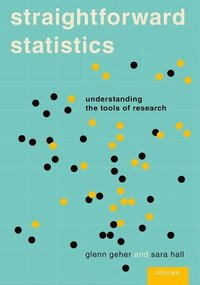## midnight run

Posted in Running, Travel with tags , , , , , , , , on December 8, 2019 by xi'an

## Le Monde puzzle [#1114]

Posted in Kids, R with tags , , , , , , on October 16, 2019 by xi'anAnother very low-key arithmetic problem as Le Monde current mathematical puzzle:

32761 is 181² and the difference of two cubes, which ones? And 181=9²+10², the sum of two consecutive integers. Is this a general rule, i.e. the root z of a perfect square that is the difference of two cubes is always the sum of two consecutive integers squared?

The solution proceeds by a very dumb R search of cubes, leading to

34761=105³-104³

The general rule can be failed by a single counter-example. Running

```sol=0;while(!sol){
x=sample(2:1e3,1)
y=sample(1:x,1)-1
sol=is.sqr(z<-x^3-y^3)
z=round(sqrt(z))
if (sol)
sol=(trunc(sqrt(z/2))^2+ceiling(sqrt(z/2))^2!=z)}
```

which is based on the fact that, if z is the sum of two consecutive integers squared, a² and (a+1)² then

2 a²<z<2 (a+1)²

Running the R code produces

x=14, y=7

as a counter-example. (Note that, however, if the difference of cubes of two consecutive integers is a square, then this square can be written as the sum of the squares of two different integers.) Reading the solution in the following issue led me to realise I had missed the consecutive in the statement of the puzzle!

## How many subjects? [not a book review]

Posted in Books, pictures, Statistics with tags , , , , , , on September 24, 2018 by xi'an

## take those hats off [from R]!

Posted in Books, Kids, R, Statistics, University life with tags , , , , , , , on May 5, 2015 by xi'anThis is presumably obvious to most if not all R programmers, but I became aware today of a hugely (?) delaying tactic in my R codes. I was working with Jean-Michel and Natesh [who are visiting at the moment] and when coding an MCMC run I was telling them that I usually preferred to code Nsim=10000 as Nsim=10^3 for readability reasons. Suddenly, I became worried that this representation involved a computation, as opposed to Nsim=1e3 and ran a little experiment:

```> system.time(for (t in 1:10^8) x=10^3)
utilisateur     système      écoulé
30.704       0.032      30.717
> system.time(for (t in 1:1e8) x=10^3)
utilisateur     système      écoulé
30.338       0.040      30.359
> system.time(for (t in 1:10^8) x=1000)
utilisateur     système      écoulé
6.548       0.084       6.631
> system.time(for (t in 1:1e8) x=1000)
utilisateur     système      écoulé
6.088       0.032       6.115
> system.time(for (t in 1:10^8) x=1e3)
utilisateur     système      écoulé
6.134       0.029       6.157
> system.time(for (t in 1:1e8) x=1e3)
utilisateur     système      écoulé
6.627       0.032       6.654
> system.time(for (t in 1:10^8) x=exp(3*log(10)))
utilisateur     système      écoulé
60.571        0.000     57.103
```

So using the usual scientific notation with powers is taking its toll! While the calculator notation with e is cost free… Weird!

I understand that the R notation 10^6 is an abbreviation for a power function that can be equally applied to pi^pi, say, but still feel aggrieved that a nice scientific notation like 10⁶ ends up as a computing trap! I thus asked the question to the Stack Overflow forum, getting the (predictable) answer that the R code 10^6 meant calling the R power function, while 1e6 was a constant. Since 10⁶ does not differ from ππ, there is no reason 10⁶ should be recognised by R as a million. Except that it makes my coding more coherent.

```> system.time( for (t in 1:10^8) x=pi^pi)
utilisateur     système      écoulé
44.518       0.000      43.179
> system.time( for (t in 1:10^8) x=10^6)
utilisateur     système      écoulé
38.336       0.000      37.860
```

Another thing I discovered from this answer to my question is that negative integers are also requesting call to a function:

```> system.time( for (t in 1:10^8) x=1)
utilisateur     système      écoulé
10.561       0.801      11.062
> system.time( for (t in 1:10^8) x=-1)
utilisateur     système      écoulé
22.711       0.860      23.098
```

This sounds even weirder.

## straightforward statistics [book review]

Posted in Books, Kids, Statistics, University life with tags , , , , , , , on July 3, 2014 by xi'an

“I took two different statistics courses as an undergraduate psychology major [and] four different advanced statistics classes as a PhD student.” G. GeherStraightforward Statistics: Understanding the Tools of Research by Glenn Geher and Sara Hall is an introductory textbook for psychology and other social science students. (That Oxford University Press sent me for review in CHANCE. Nice cover, by the way!) I can spot the purpose behind the title, purpose heavily stressed anew in the preface and the first chapter, but it nonetheless irks me as conveying the message that one semester of reasonable diligence in class will suffice to any college students to “not only understanding research findings from psychology, but also to uncovering new truths about the world and our place in it” (p.9). Nothing less. While, in essence, it covers the basics found in all introductory textbooks, from descriptive statistics to ANOVA models. The inclusion of “real research examples” in the chapters of the book rather demonstrates how far from real research a reader of the book would stand… Continue reading